Dynamic Oracles for Top-Down and In-Order Shift-Reduce Constituent Parsing# 介绍

Dynamic Oracle是用在转移系统中，防止错误传播的一种手段。而转移系统主要有分为三种：bottom-up、top-down和in-order的转移系统。

# 基础知识

## 形式化定义

bottom-up的转移系统这里就不讨论了，这里主要讨论另外两种转移系统。转移系统的状态用五元组$c = \left\langle {\sum ,i,f,\gamma ,\alpha } \right\rangle$表示，五元组内元素分别表示stack、buffer第一个单词的下标、in-order转移系统中结束标记、已经生成的短语成分集合、stack中非终结符集合。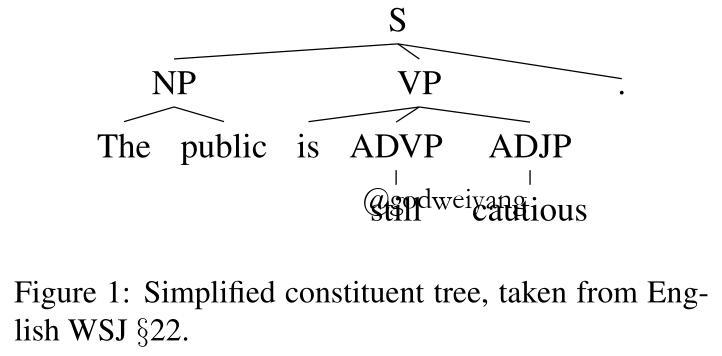$(S,0,6),(NP,0,2),(VP,2,5),(ADVP,3,4),(ADJP,4,5)$如果采用top-down的转移系统，非终结符入栈的顺序为
$(S, 0), (NP, 0), (VP, 2), (ADVP, 3), (ADJP, 4)$如果采用in-order的转移系统，非终结符入栈的顺序为
$(NP, 1), (S, 2), (VP, 3), (ADVP, 4), (ADJP, 5)$

## top-down转移系统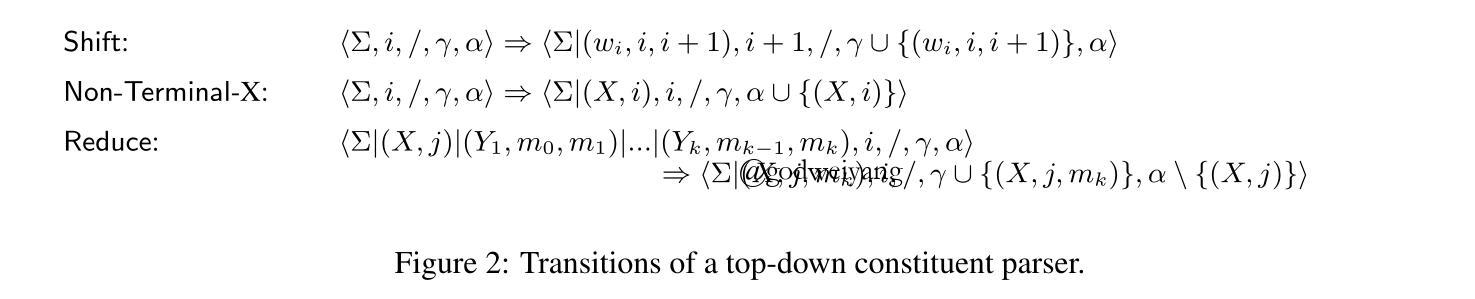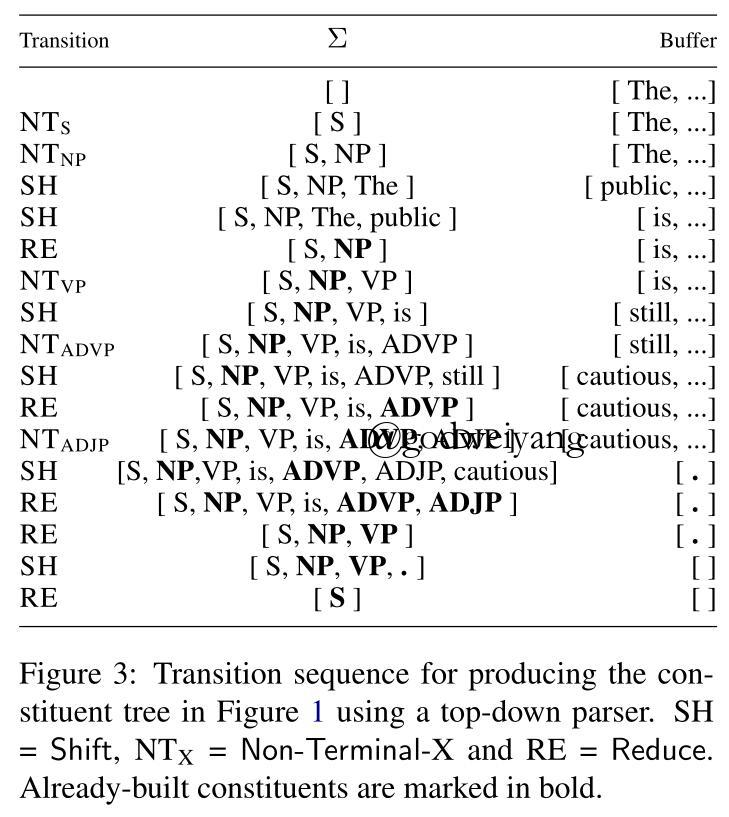## in-order转移系统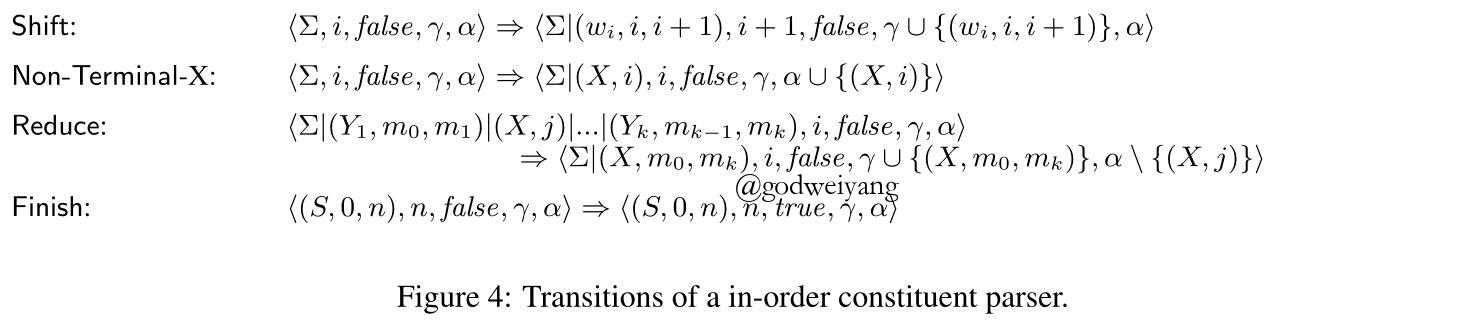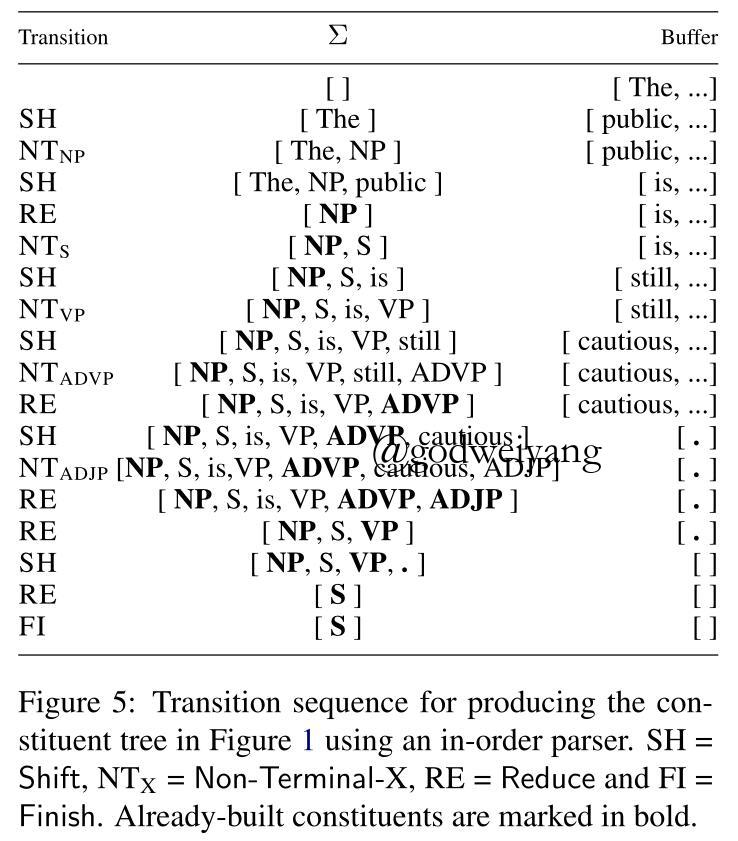# Dynamic Oracles

Goldberg (2012)证明了Dynamic Oracle可以通过定义一个损失函数来直接实现，而这个损失函数可以用来衡量当前状态可以产生的最优句法树和标准句法树的距离。最小化这个距离就会使得错误状态也会转移到最终错误最少的状态。而这个损失函数就要和当前状态c挂钩了，这样才能达到和传统的Dynamic Oracle类似的效果。

## 损失函数

$\mathcal{l}(c) = \min_{\gamma | c \to \gamma} \mathcal{L}(\gamma, \gamma_G) = \left| { {\gamma _G}\backslash \gamma } \right| + \left| {\gamma \backslash {\gamma _G}} \right|$

$\mathcal{l}(c) = \min_{\gamma | c \to \gamma} \mathcal{L}(\gamma, \gamma_G) = \left| \mathcal{U}(c, \gamma_G) \right| + \left| {\gamma_c \backslash {\gamma _G}} \right|$

• 当前栈中已经生成的non-terminal集合$\alpha_c$中不包含在标准non-terminal集合$\alpha_G$中的non-terminal数量，即$\left| \alpha_c \backslash \alpha_G \right|$。
• 当前栈中违反了标准树中non-terminal顺序的non-terminal数量。

$\mathcal{l}(c) = \min_{\gamma | c \to \gamma} \mathcal{L}(\gamma, \gamma_G) = \left| \mathcal{U}(c, \gamma_G) \right| + \left| {\gamma_c \backslash {\gamma _G}} \right| + \left| \alpha_c \backslash \alpha_G \right| + out \_ of \_ order(\alpha_c, \alpha_G)$

## 短语的可达性

• $(X, l, r) \in \gamma_c$（因为短语已经包含在了状态c已生成的短语集合里，那么它当然是可达的）。
• $j \le l < r \wedge (X, l) \notin \alpha_c$（因为短语还在buffer中，并且短语的non-terminal还没有入栈，所以可以通过入栈$(X, l)$，再不断SHIFT然后REDUCE得到）。
• $l \in \{i_k | 1 \le k \le p\} \wedge j \le r \wedge (X, l) \in \alpha_c$（这种情况表明了短语的左端点恰好位于栈里某个短语的边界处，而右端点又还在buffer里，所以还可以通过不断SHUFT然后REDUCE得到短语。但是如果左端点不是栈里短语的边界，那说明产生了交叉，自然不会可达了。而如果右端点已经在栈里了，那之后也不会得到了，因为转移系统每次都是REDUCE栈顶的短语，不可能从栈里面开始REDUCE的，当然这些前提条件当然是non-terminal$(X, l)$已经在栈里了）。

• $(X, l, r) \in \gamma_c$（因为短语已经包含在了状态c已生成的短语集合里，那么它当然是可达的）。
• $j \le l < r$（因为短语还在buffer中，所以可以通过入栈第一个左儿子，再入栈$(X, m)$，再不断SHIFT然后REDUCE得到）。
• $l \in \{i_k | 1 \le k \le p\} \wedge j \le r \wedge (X, m) \notin \alpha_c$（这种情况表明了第一个左儿子已经生成了一部分或者完全生成了，并且根结点non-terminal还没有入栈，所以依然可以生成）。
• $l \in \{i_k | 1 \le k \le p\} \wedge j \le r \wedge (X, m) \in \alpha_c \wedge \exists (Y,l,m) \in \sum$（这种情况表明了第一个左儿子已经完全生成了，并且根结点non-terminal在栈里，所以依然可以生成）。

# 实验结果转载规则

《Dynamic Oracles for Top-Down and In-Order Shift-Reduce Constituent Parsing》 韦阳 采用 知识共享署名 4.0 国际许可协议 进行许可。
上一篇二零一八年终总结

2018-12-31Faster Shift-Reduce Constituent Parsing with a Non-Binary, Bottom-Up Strategy

2018-11-06
目录